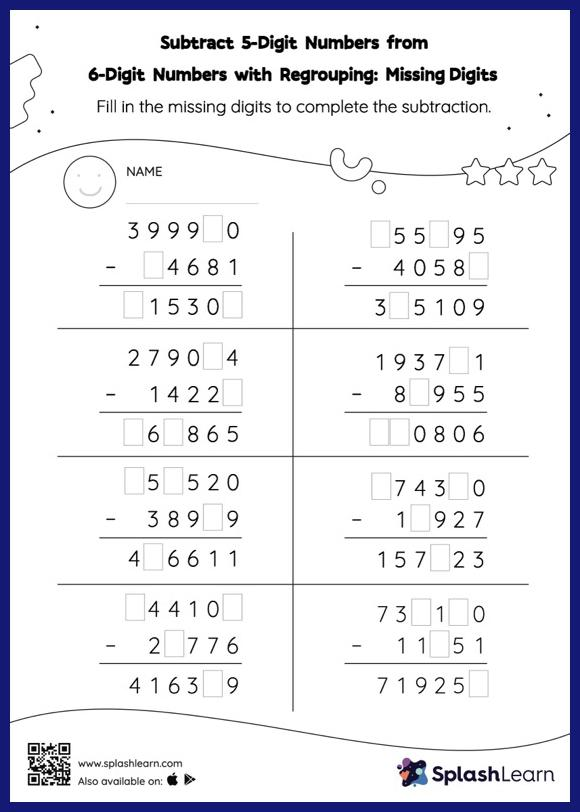# Subtract 5-Digit Numbers from 6-Digit Numbers with Regrouping: Missing Digits Worksheet

Home > Subtract 5-Digit Numbers from 6-Digit Numbers with Regrouping: Missing DigitsLearners hone their math skills with this subtract 5-digit numbers from 6-digit numbers with regrouping worksheet. In this worksheet, students must find the missing number using the count back method or the relationship between addition and subtraction. To solve subtract 5-digit numbers from 6-digit numbers with regrouping worksheet, they must also regroup the numbers. This worksheet is about practicing the column method in which numbers are written one on top of another. In this method, students use their place value understanding to solve the problems.